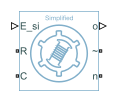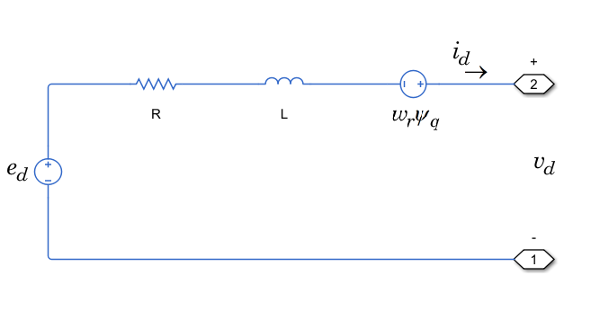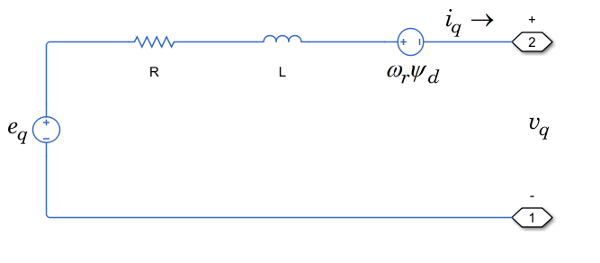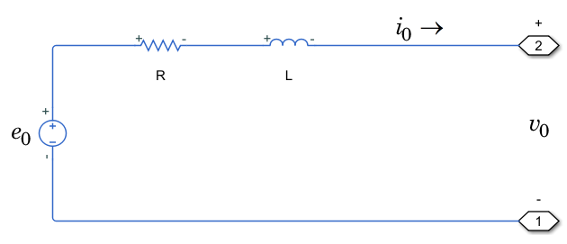# Simplified Synchronous Machine

Simplified synchronous machine with electromotive force

• Library:
• Simscape / Electrical / Electromechanical / Synchronous

•## Description

The Simplified Synchronous Machine block models a simplified synchronous machine with a voltage source that represents electromotive force (EMF). You can specify the internal resistance and inductance with per-unit or SI parameters.

The equivalent circuits of the simplified synchronous machine for the direct axis, the quadrature axis, and the zero sequence are:### Equations

The simplified synchronous machine equations are expressed with respect to a rotating reference frame, which is defined by:

`${\theta }_{e}\left(t\right)=N{\theta }_{r}\left(t\right),$`

where:

• θe is the electrical angle.

• N is the number of pole pairs.

• θr is the rotor angle.

The Park transformation maps the synchronous machine equations to the rotating reference frame with respect to the electrical angle. The Park transformation is defined by:

`${P}_{s}=\frac{2}{3}\left[\begin{array}{ccc}-\mathrm{cos}{\theta }_{e}& -\mathrm{cos}\left({\theta }_{e}-\frac{2\pi }{3}\right)& -\mathrm{cos}\left({\theta }_{e}+\frac{2\pi }{3}\right)\\ \mathrm{sin}{\theta }_{e}& \mathrm{sin}\left({\theta }_{e}-\frac{2\pi }{3}\right)& \mathrm{sin}\left({\theta }_{e}+\frac{2\pi }{3}\right)\\ \frac{1}{2}& \frac{1}{2}& \frac{1}{2}\end{array}\right].$`

The Park transformation is used to define the per-unit simplified synchronous machine equations. The voltage equations are defined by:

`${e}_{d}=\frac{1}{{\omega }_{base}}\frac{\text{d}{\psi }_{d}}{\text{d}t}-{\Psi }_{q}{\omega }_{r}+R{i}_{d}+{v}_{d}$`

`${e}_{q}=\frac{1}{{\omega }_{base}}\frac{\text{d}{\psi }_{q}}{\text{d}t}+{\Psi }_{d}{\omega }_{r}+R{i}_{q}+{v}_{q}$`

`${e}_{0}=\frac{1}{{\omega }_{base}}\frac{d{\Psi }_{0}}{dt}+R{i}_{0}+{v}_{0}$`

where:

• ed, eq, and e0 are the d-axis, q-axis, and zero-sequence voltages, defined by:

`$\left[\begin{array}{c}{e}_{d}\\ {e}_{q}\\ {e}_{0}\end{array}\right]={P}_{s}\left[\begin{array}{c}{e}_{a}\\ {e}_{b}\\ {e}_{c}\end{array}\right].$`

ea, eb, and ec are the per-unit internal voltage sources, defined by:

`$\begin{array}{l}{e}_{a}={E}_{pu}sin{\theta }_{e}\\ {e}_{b}={E}_{pu}sin\left({\theta }_{e}-120\text{°)}\\ {\text{e}}_{c}={E}_{pu}sin\left({\theta }_{e}+120\text{°)}\end{array}$`

epu is the per-unit amplitude of the internal generated voltage.

• vd, vq, and v0 are defined by:

`$\left[\begin{array}{c}{v}_{d}\\ {v}_{q}\\ {v}_{0}\end{array}\right]={P}_{s}\left[\begin{array}{c}{v}_{a}\\ {v}_{b}\\ {v}_{c}\end{array}\right].$`

va, vb, and vc are the stator voltages measured from port ~ to neutral port n.

• ωbase is the per-unit base electrical speed.

• ψd, ψq, and ψ0 are the d-axis, q-axis, and zero-sequence flux linkages.

• ωr is the per-unit rotor rotational speed.

• R is the stator resistance.

• id, iq, and i0 are the d-axis, q-axis, and zero-sequence stator currents, defined by:

`$\left[\begin{array}{c}{i}_{d}\\ {i}_{q}\\ {i}_{0}\end{array}\right]={P}_{s}\left[\begin{array}{c}{i}_{a}\\ {i}_{b}\\ {i}_{c}\end{array}\right].$`

ia, ib, and ic are the stator currents flowing out of port .

The stator flux linkage equations are defined by

`$\begin{array}{l}{\psi }_{d}=L\cdot {i}_{d}\\ {\psi }_{q}=L\cdot {i}_{q}\\ {\psi }_{0}=L\cdot {i}_{0}\end{array}$`

where L is the stator leakage inductance.

The power equation of the simplified synchronous machine in per-unit is defined by:

`$P={e}_{d}{i}_{d}+{e}_{q}{i}_{q}.$`

### Plotting and Display Options

You can perform plotting and display actions using the Electrical menu on the block context menu.

Right-click the block and, from the Electrical menu, select one of these options:

• Display Base Values — Displays the machine per-unit base values in the MATLAB® Command Window.

• Display Associated Initial Conditions — Displays associated initial conditions in the MATLAB Command Window.

### Model Thermal Effects

You can expose thermal ports to model the effects of generated heat and machine temperature. To expose the thermal ports, set the Modeling option parameter to either:

• `No thermal port` — The block does not contain thermal ports.

• `Show thermal port` — The block contains multiple thermal conserving ports.

For more information about using thermal ports in actuator blocks, see Simulating Thermal Effects in Rotational and Translational Actuators.

### Variables

To set the priority and initial target values for the block variables prior to simulation, use the Initial Targets section in the block dialog box or Property Inspector. For more information, see Set Priority and Initial Target for Block Variables.

For this block, the Initial Targets settings are visible only if, in the Initial Conditions section, you set the Initialization option parameter to ```Set targets for rotor angle and Park's transform variables```.

Nominal values provide a way to specify the expected magnitude of a variable in a model. Using system scaling based on nominal values increases the simulation robustness. Nominal values can come from different sources, one of which is the Nominal Values section in the block dialog box or Property Inspector. For more information, see System Scaling by Nominal Values.

## Ports

### Input

expand all

Physical signal port associated with the amplitude of the internal generated voltage (phase-to-neutral), specified as a vector of physical signals.

#### Dependencies

To enable this port, set Parameterization unit to `SI`.

Physical signal port associated with the per-unit amplitude of the internal generated voltage, specified as a vector of physical signals.

#### Dependencies

To enable this port, set Parameterization unit to `Per unit`.

### Output

expand all

Physical signal port associated with the machine per-unit measurements, returned as a vector of physical signals. The vector elements are:

• Electromotive force associated with phase a, pu_EMF(1)

• Electromotive force associated with phase b, pu_EMF(2)

• Electromotive force associated with phase c, pu_EMF(3)

• Electrical torque, pu_torque

• Rotor velocity, pu_velocity

• Stator `d`-axis voltage, pu_vd

• Stator `q`-axis voltage, pu_vq

• Stator zero-sequence voltage, pu_e0

• Stator `d`-axis current, pu_id

• Stator `q`-axis current, pu_iq

• Stator zero-sequence current, pu_i0

• Rotor electrical angle, electrical_angle_out

To connect to this port, use the Synchronous Machine Measurement block.

### Conserving

expand all

Mechanical rotational conserving port associated with the machine rotor.

Mechanical rotational conserving port associated with the machine case.

Expandable three-phase electrical port associated with the stator windings.

#### Dependencies

To enable this port, set Electrical connection to ```Composite three-phase ports```.

Electrical conserving port associated with the neutral point of the wye winding configuration.

Electrical conserving port associated with a-phase.

#### Dependencies

To enable this port, set Electrical connection to ```Expanded three-phase ports```.

Electrical conserving port associated with b-phase.

#### Dependencies

To enable this port, set Electrical connection to ```Expanded three-phase ports```.

Electrical conserving port associated with c-phase.

#### Dependencies

To enable this port, set Electrical connection to ```Expanded three-phase ports```.

Thermal conserving port associated with stator winding a.

#### Dependencies

To enable this port, set Modeling option to `Show thermal port`.

Thermal conserving port associated with stator winding b.

#### Dependencies

To enable this port, set Modeling option to `Show thermal port`.

Thermal conserving port associated with stator winding c.

#### Dependencies

To enable this port, set Modeling option to `Show thermal port`.

Thermal conserving port associated with the rotor.

#### Dependencies

To enable this port, set Modeling option to `Show thermal port`.

## Parameters

expand all

Whether to enable the thermal ports of the block and model the effects of generated heat and machine temperature.

### Main

Whether to have composite or expanded three-phase ports.

Rated apparent power.

RMS rated line-to-line voltage.

Nominal electrical frequency at which rated apparent power is quoted.

Number of machine pole pairs.

Block parameterization method. Options are:

• `SI`

• `Per unit`

Internal per-unit resistance.

#### Dependencies

To enable this parameter, set Parameterization unit to `Per unit`.

Internal per-unit inductance in per-unit.

#### Dependencies

To enable this parameter, set Parameterization unit to `Per unit`.

Internal resistance.

#### Dependencies

To enable this parameter, set Parameterization unit to `SI`.

Internal inductance.

#### Dependencies

To enable this parameter, set Parameterization unit to `SI`.

### Initial Conditions

Model for specifying values for certain parameters and variables at the start of simulation. To:

• Set an operating point regardless of the connected network, select ```Set real power, reactive power, terminal voltage and terminal phase```.

• Specify the priority and initial target values for block variables before simulation using the Variables settings, select ```Set targets for rotor angle and Park's transform variables```. For more information, see Set Priority and Initial Target for Block Variables.

• Select a bus type and specify the related parameters for a load-flow analysis in the Initial Conditions settings, select ```Set targets for load flow variables```.

#### Dependencies

If you set this parameter to:

• ```Set targets for rotor angle and Park's transform variables``` — The Variables settings become visible.

• ```Set real power, reactive power, terminal voltage, and terminal phase``` — Related parameters become visible.

• ```Set targets for load flow variables``` — Related parameters become visible.

Type of voltage source that the block models.

#### Dependencies

To enable this parameter set Initialization option to ```Set targets for load flow variables``` and Source type to `Swing bus` or ```PV bus```.

Terminal voltage magnitude.

#### Dependencies

To enable this parameter, set Initialization option to ```Set real power, reactive power, terminal voltage, and terminal phase``` or Initialization option to ```Set targets for load flow variables``` and Source type to ```Swing bus``` or `PV bus`.

Terminal voltage angle.

#### Dependencies

To enable this parameter, set Initialization option to ```Set real power, reactive power, terminal voltage, and terminal phase``` or Initialization option to ```Set targets for load flow variables``` and Source type to ```Swing bus```.

Active power generated.

#### Dependencies

To enable this parameter, set Initialization option to ```Set real power, reactive power, terminal voltage, and terminal phase``` or Initialization option to ```Set targets for load flow variables``` and Source type to ```PV bus``` or `PQ bus`.

Reactive power generated.

#### Dependencies

To enable this parameter, set Initialization option to ```Set real power, reactive power, terminal voltage, and terminal phase``` or Initialization option to ```Set targets for load flow variables``` and Source type to ```PQ bus```.

#### Dependencies

To enable this parameter, set Initialization option to ```Set targets for load flow variables``` and Source type to `PQ bus`.

Vector that defines the phase angle search range.

#### Dependencies

To enable this parameter, set Initialization option to ```Set targets for load flow variables``` and Source type to `PV bus` or ```PQ bus```.

Parasitic conductance to the electrical reference.

#### Dependencies

To enable this parameter, set Initialization option to ```Set targets for load flow variables```.

### Thermal

To enable these parameters, set Modeling option to `Show thermal port`.

Temperature for which motor parameters are quoted.

Coefficient α in the equation relating resistance to temperature for all three windings, as described in Thermal Model for Actuator Blocks. The default value, `3.93e-3` 1/K, is for copper.

Thermal mass value for each stator winding. The thermal mass is the energy required to raise the temperature by one degree.

Thermal mass of the rotor. The thermal mass is the energy required to raise the temperature of the rotor by one degree.

## Version History

Introduced in R2020b

expand all